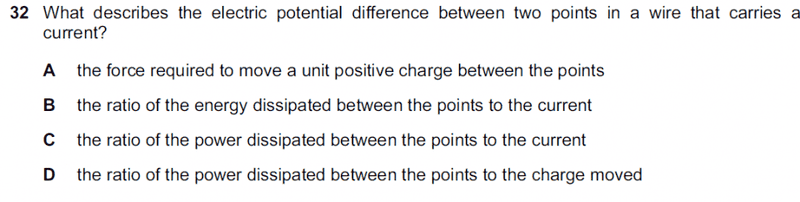# Description of electric potential difference?

## Homework StatementV=IR
W=VQ

## The Attempt at a Solution

The answer is B, though I thought that electric potential difference is the ratio of energy to charge? Why is the answer stating the ratio of energy to current instead?

## Answers and Replies

Are you sure the answer is B ?

Are you sure the answer is B ?
Are you sure the answer is B ?
Oops, so very sorry. Read the wrong answer. Answer is C. Sorry again